Name:_______________________ ENS 495 Fall 2016 12/15/2016 Test 3: Regression

#This is the test key.
#Notes regarding the test will be sent via email

#COrrect answers are flagged with the text
# "**CORRECT**"

All questions are weighted as 2 points unless otherwise stated.

Question 1: Which TWO statements in the list below about correlation analysis are TRUE and can be used to complete the following sentence:

Correlation analysis investigates/determine if… (circle 2 answers)

1. There is a significant relationship between 2 variables CORRECT!
2. The relationship between variables is positive or negative CORRECT!
3. The relationship between 3 or more variables is significant
4. The relationship between a binomial variable and a numeric variable is significant.
5. One variable is physically or biological causing change in another

Question 2: Regression analysis is different than correlation analysis for which of the following reasons (that is, what does regression analysis assume or do that correlation analysis does not or can not?)

#This question should be re-worded if used in teh future
1. It can determine if there is a positive or negative relationship between y and x.
2. It assumes no causal relationship between y and x.
3. It cannot be used for prediction
4. It assumes a directional relationship between variables, such as y is caused by x. CORRECT!

Question 3: What mathematical method is used to fit a regression line to data?

1. Maximum Entropy
2. Least Squares CORRECT!
3. Correlation analysis
4. Mean square error (MSE)
5. All of the above
6. None of the above

Question 4: When you fit a regression line to data, the TWO parameters that describe form of the line through the scatterplot are: (circle 2 answers)

1. Slope CORRECT!
2. p-value
3. F-statistic
4. Standard error (SE)
5. R^2
6. Intercept CORRECT!

Question 5: When you run a regression, uncertainty about the true value of the slope of the line can be characterized

1. p-value for the regression model
2. Standard error (SE) and/or confidence interval (CI) CORRECT!
3. F-statistics for the model
4. R^2 value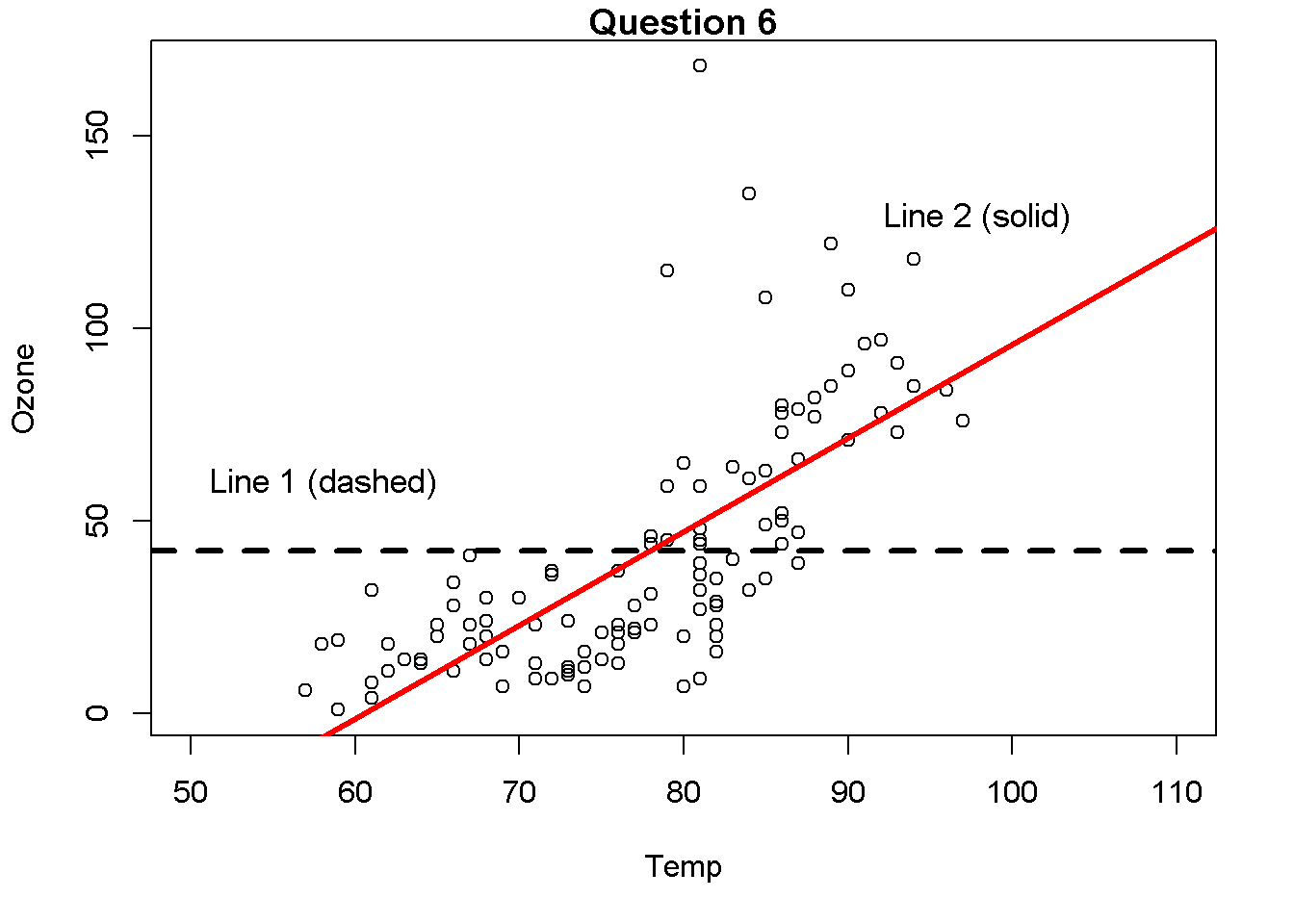Question 6 For the following questions consider the graph above

6a: Which line in the graph above represents the NULL Hypothesis or model (Ho)? (1 point)

1. Line 1 (Flat dashed line) CORRECT!
2. Line 2 (Angled solid line)

6b: For the graph above, which line represents the Alternative Hypothesis or model (Ha)? (1 point)

1. Line 1 (Flat dashed line)
2. Line 2 (Angled solid line) CORRECT!

6c) Just looking at the graph, which hypothesis do you think is most likely to be true? (1 point)

1. Null hypothesis (Ho)
2. Alternative hypothesis (Ha) CORRECT!

6d) What term best characterizes Line 1

1. Positive slope (+)
2. Negative slope (-)
3. Slope of zero (0) CORRECT!
4. The term “slope” is not relevant

6e) What is the “word equation” that would be appropriate for a regression model for these data in the plot?

1. Ozone ~ Intercept + Slope*Temp CORRECT!
2. Temp ~ Intercept + Ozone*Temp
3. Ozone ~ Temp
4. y ~ mx + b

Question 7) A model using temp and ozone was fit to these data usig the lm() function in R. The following output was produced using the summary() command.

Estimate Std. Error t value Pr(>|t|)

Intercept -146.9955 18.2872 -8.038 9.37e-13 Temp 2.4287 0.2331 10.418 < 2e-16

7a) Write the full mathematical equation described by this output.

7b) In the output above, what is the p-value? Write it below.

7c) How do you interpret this p-value.

1. Significant
2. Non-significant
3. Marginally significant
4. Not enough information
5. p-values are dumb

Question 8) TRUE / FALSE (circle one) Regression, ANOVA and t-tests are all fundamentally different methods that require different statistical approaches. (1 point)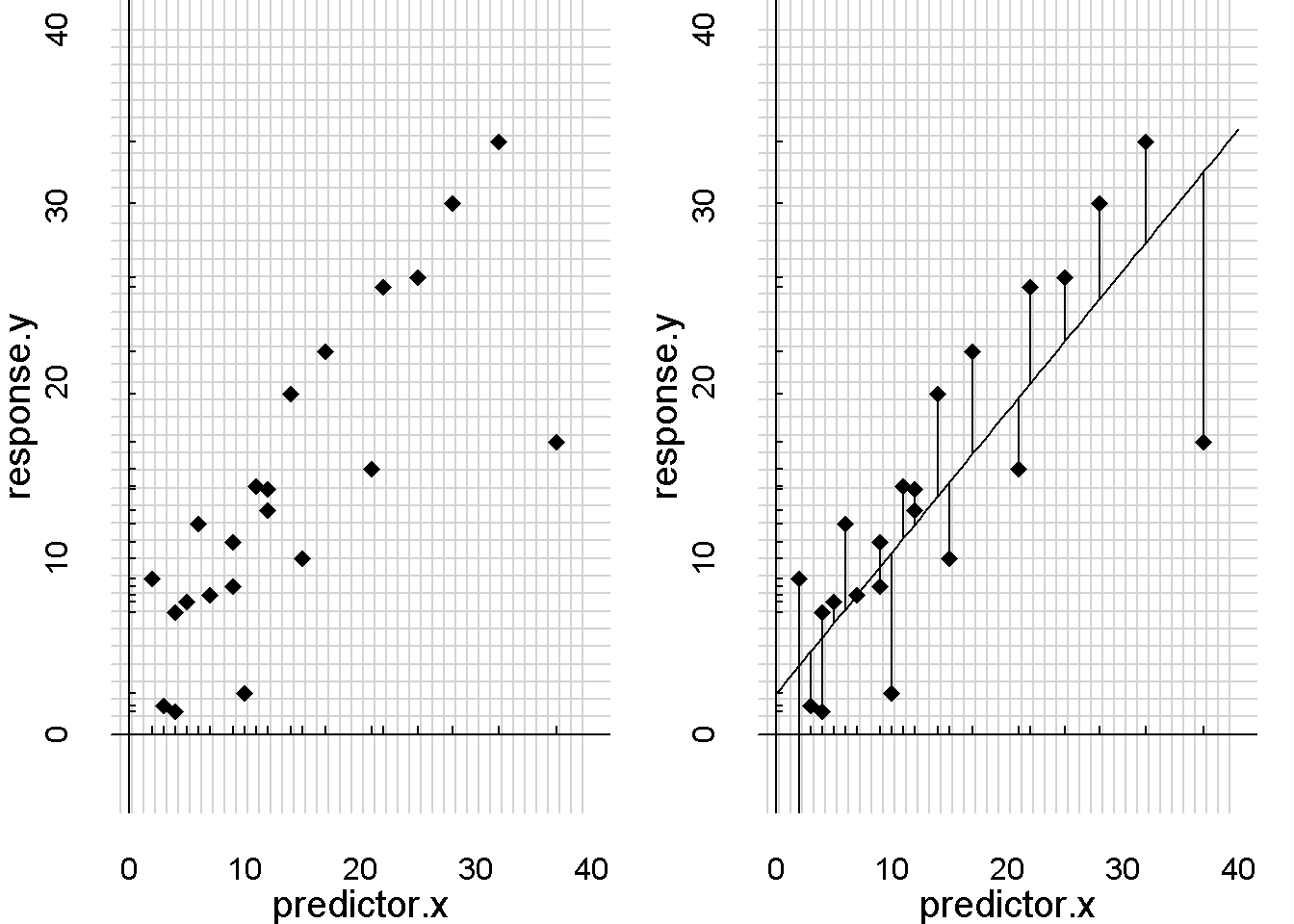Question 9) In the figure above, the left-hand size of the plot shows a scatter plot of the data. The right hand side shows a regression line through the data.

What are the vertical lines drawn from the regression line up or down to each data point?

1. Residuals
2. Intercepts
3. Slopes
4. Least squares
5. Mean square errors (MSE)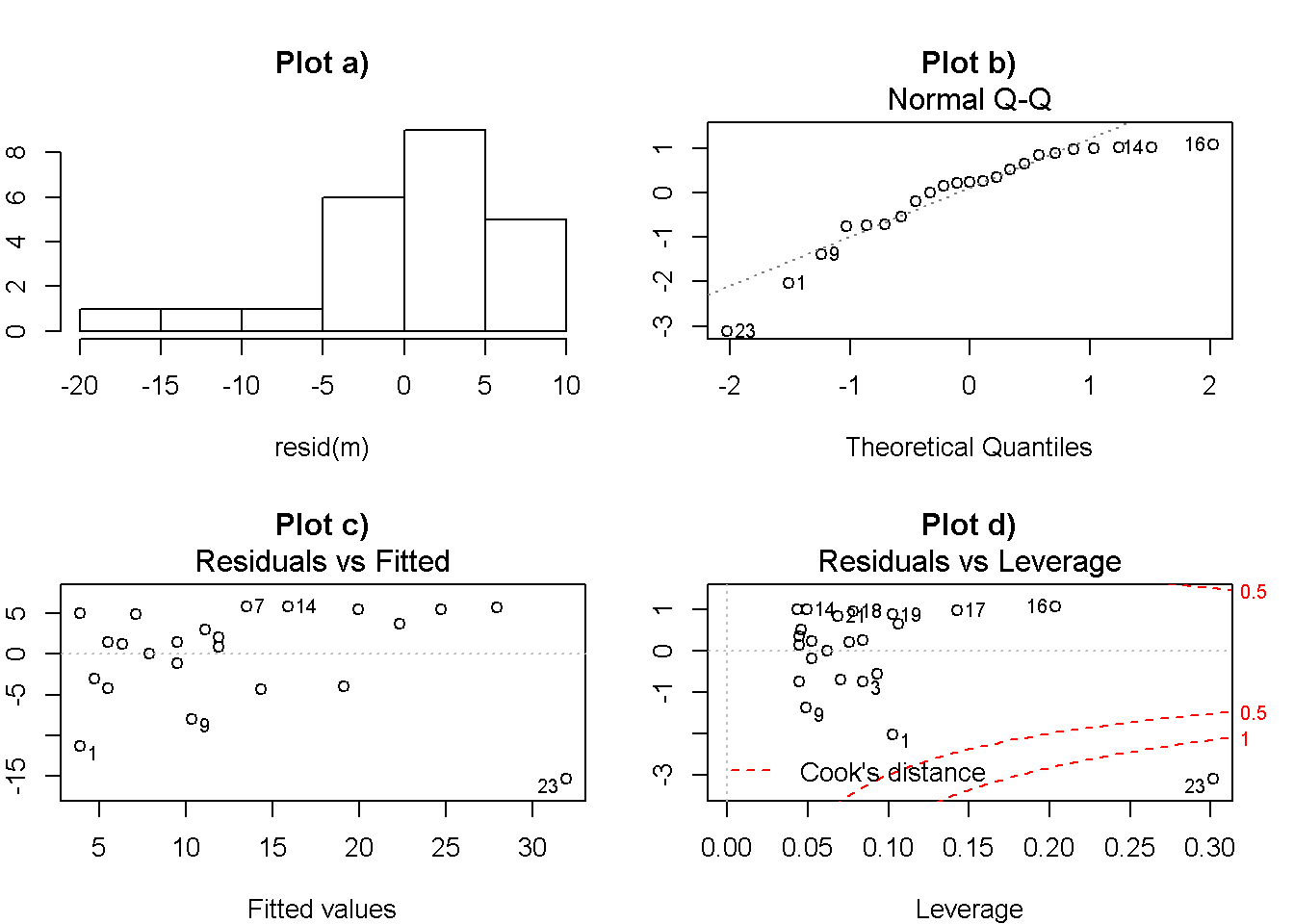Question 10) The above plot show diagnostics from the scatter plot in the previous question.

10a) Which TWO plots provide information on the normality of the data? (circle 2 answers)

1. Plot a
2. Plot b
3. Plot c
4. Plot e

10b) Which plot provides information about whether there are any outliers and/or influental points in the data set?

1. Plot a
2. Plot b
3. Plot c
4. Plot e

10c) Is there a outer/influential point in this data set, and if so what is it? (1 point)

1. There is not an outlier/influential point
2. There is an influential point and it is _________

Question 11: When doing regression, the process involves calculating the residuals of the regression model and doing what mathematically to them to determine the line that best fits the data?

1. Doing nothing to them. The line of best fit is determined using the raw residuals
2. Getting rid of the negative sign (-) by taking the absolute value (abs) of the residual
3. Getting rid of the negative sign (-) by squaring the residual (eg, residual^2)
4. Cubing the residuals, ie I(residual^3)

Question 12: The standard error (SE) of the slope of a regression line represents:

1. Variation in the slope due to non-random sampling
2. Error due to non-normal residuals
3. Uncertainty about the true slope of the line
4. Variation in the intercept
5. All of the above
6. None of the above

Question 13: The value of R^2 from a regression tells us:

1. How well the model fits the data
2. If the line is significantly different from zero
3. If the p-value is small
4. If the slope is positive or negative
5. None of the above
6. All of the above

Question 14: TRUE / FALSE: If you have a very low p-value (highly significant difference), you you must also have a very high R^2 value. Therefore, R^2 is highly correlated with p-values. (1 point)

Question 15: What are the key assumptions of regression? (4 points)

Question 16: Which of your 4 key assumptions is the most important to pay attention to (1 point; refer to to the list you made for question 17)

1. Assumption 1
2. Assumption 2
3. Assumption 3
4. Assumption 4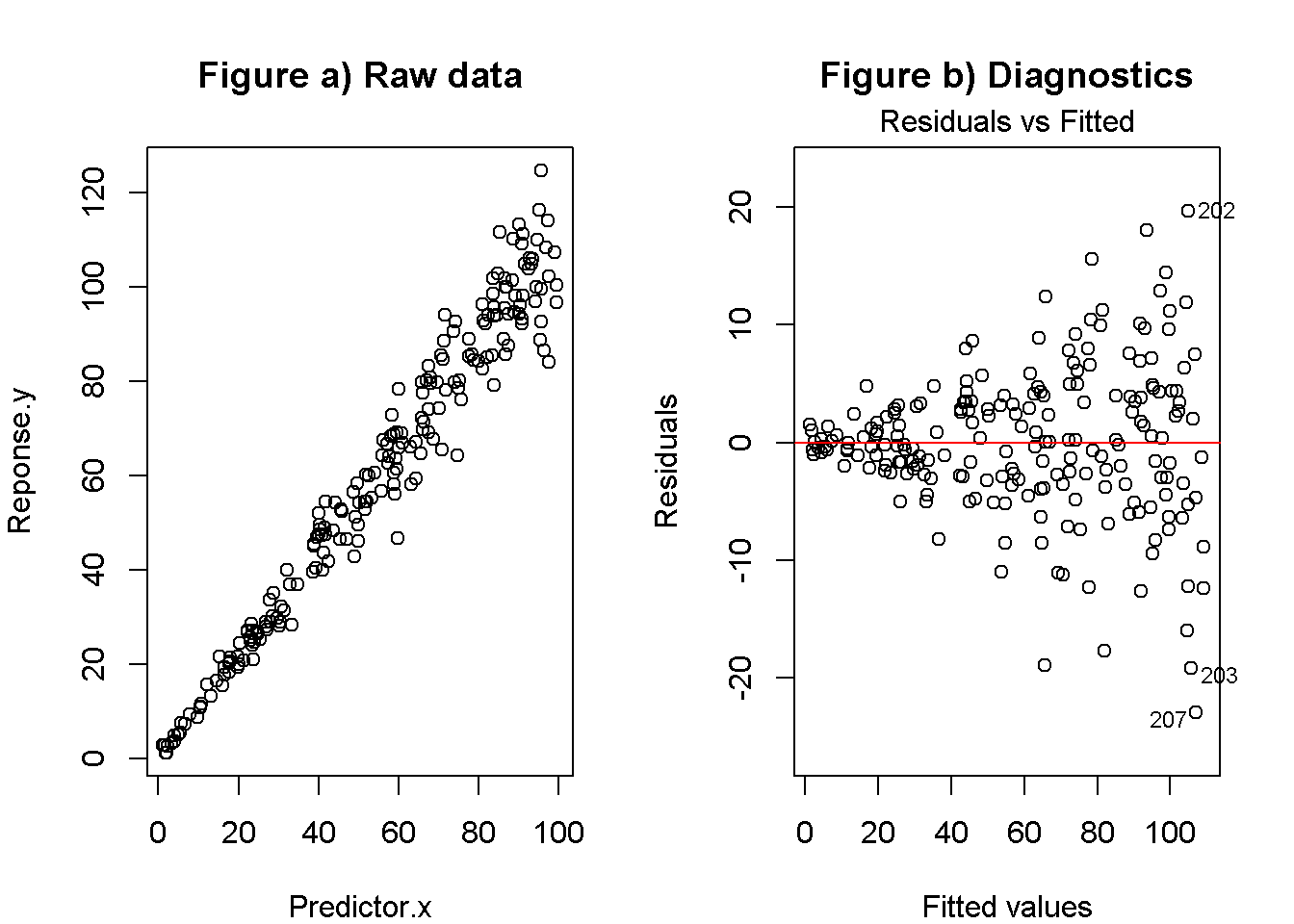Question 17: The left-hand graph above shows a plot raw data, and the right hand graph shows a diagnostic plot.

17a) What assumption of regression analysis does this diagnostics plot tell us about? (refer to the list you made above)

1. Assumption 1
2. Assumption 2
3. Assumption 3
4. Assumption 4

17b) Do these data appear to violate this assumption?

1. Yes, assumption violated.
2. No

17c) Assuming these data do indeed violate this assumption, what could be done to try to fix the problem?

1. Nothing
2. Remove outliers
3. Log transformation of predictor (x)
4. Log transformation of response (y)
5. Collect more data

Question 18: Which of the following things does the log tranformation NOT do

1. Improve normality of the residuals
2. Make the variance more constant
3. Reduce impact of non-random sampling
4. Reduce the impact of outliers

Question 19) Which two statements are true about outliers

1. All outliers are bad data points that should be removed
2. Regression models assume that there are no outliers
3. All outliers occur due to errors during data collection or data entry.
4. Diagnostics techniques such as plot cannot identify outliers
5. Outliers can occur due to real but extreme observations

x <- runif(100,1,10)
y <- 10.0 + 12.0*x + -0.872*x^2 + -0.0072*x^3 + -0.000972*x^4 + rnorm(length(x),0,10)

scatter.smooth(x,y, main = "Question 20")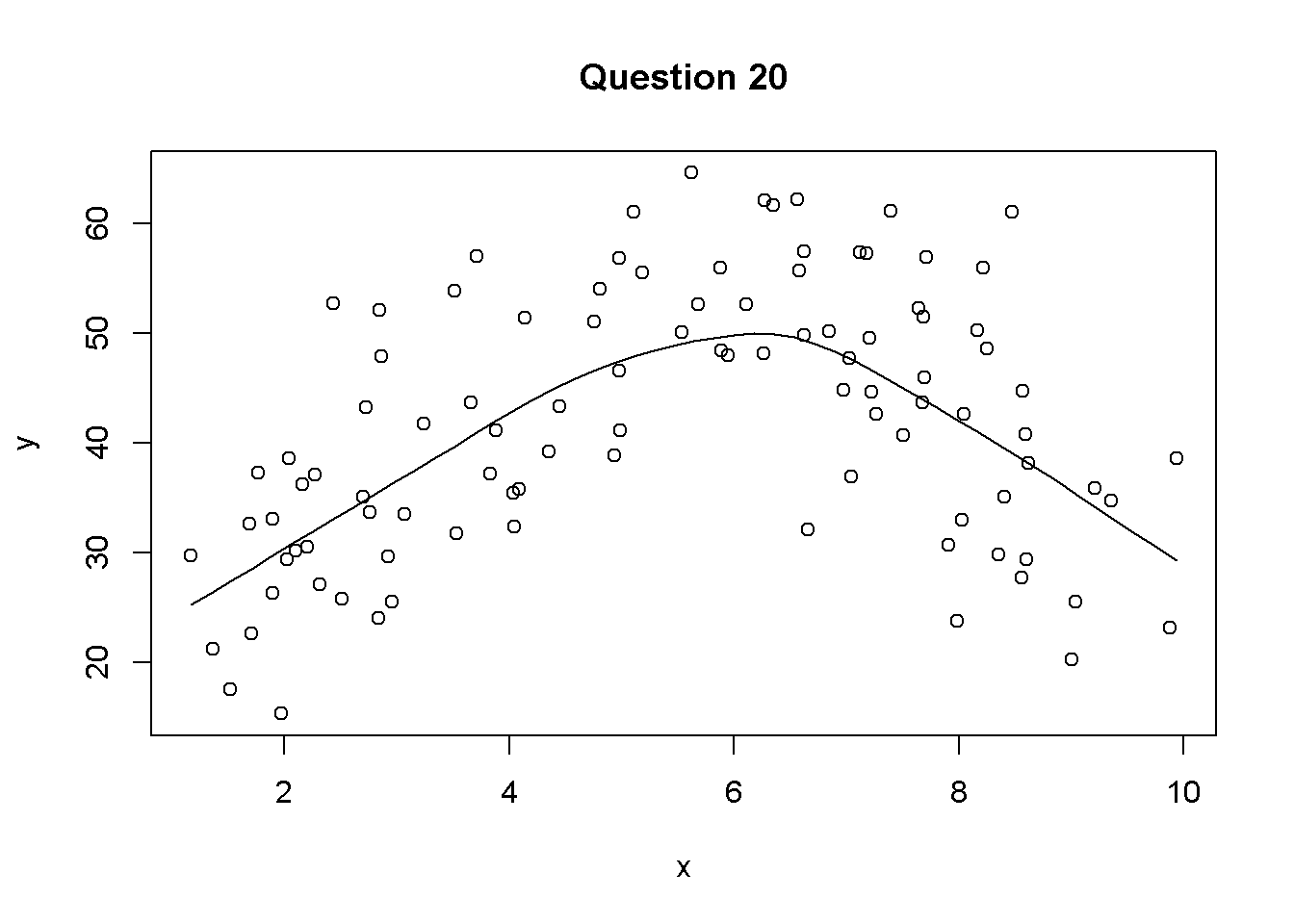Question 20) For the following questions consider this scatter plot of variable y plotted against variable x.

20a) The line through the data was not draw using regression but instead using a technique use to help visualize curvey data. What is the name of this type of line? (1/2 point)

1. A curvey line
2. Logistic model
3. 2-way ANOVA (aka 2 x 2 ANOVA)
4. A smoother
5. None of the above
6. All of the above.

20b) What is the technical name for a such as this that is not straight? (1/2 point)

1. Logistic
2. Curvey
3. Residual
4. Asymptote
5. Non-linear

Question 21) What R code would you add to a regression to model a curvey line? Assume the predictor is called “x” (1 point)

1. I
2. I()
3. I(x)
4. I(x^2)
5. I(x^3)
6. I(1/x)
7. none of the above

Question 22) Logistic regression is used to model

1. Binomial predictors
2. Categorical response w/2+ levels (red, blue, green)
3. Numeric responses
5. Numeric predictors
6. None of the above
7. All of the above

Question 23) Which of these TWO statements are true about logistic regression (circle 2)

1. Confidence intervals cannot be greater than 1
2. Confidence intervals cannot be less than 0
3. Confidence intervals cannot be calculated
4. Confidence intervals should be calculated from percentages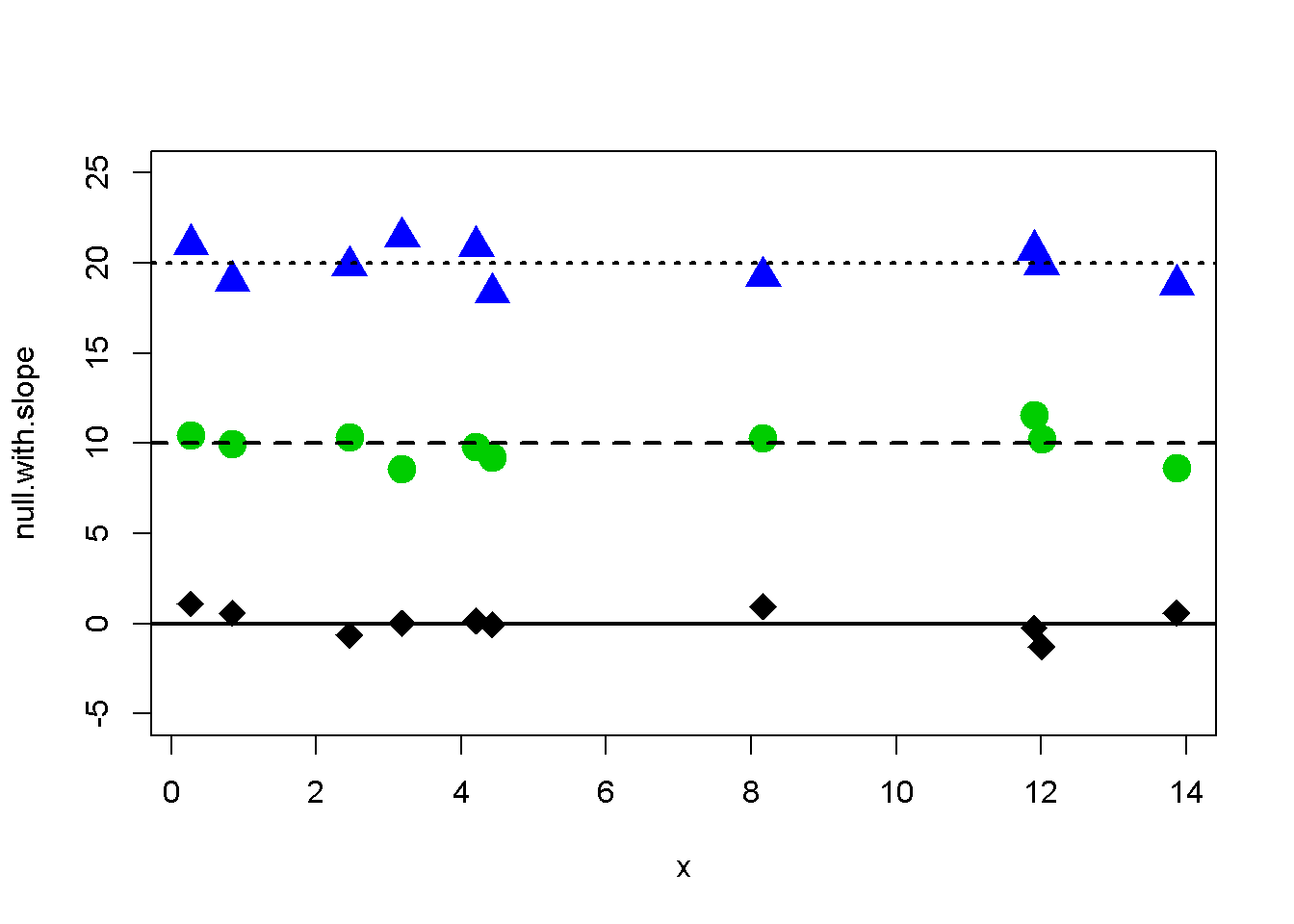Question 24) This plot shows three sets of data (diamonds, circles, triangles) and regression lines running through them. What statement is true about these lines?

1. They all have the same intercept
2. They all have the same slope
3. They are all all significantly different from 0
4. They all represent an alternative hypothesis.
5. They are all random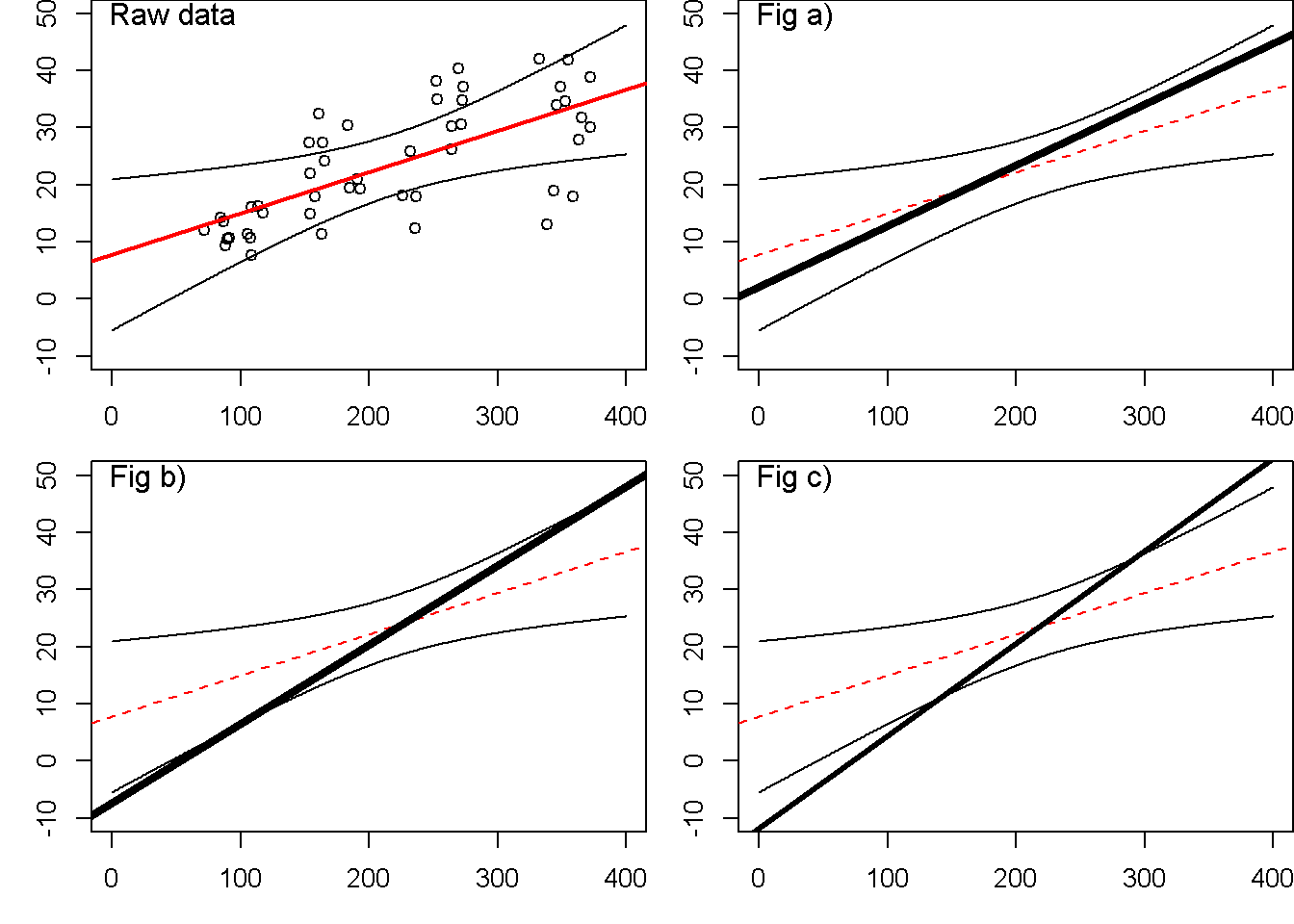Question 25) The figure above, in the top-left panel, shows scatter plot of raw data with a regression line and a “confidence band” (aka “confidence ellipse”) around the line. This line represents uncertainty about the true values of the parameters that define the line. In panels a, b, and c are shown 3 other possible regression lines as thick lines plotted with the original regression line (now thin and dotted) and confidence band.

Of the 3 alternative regression lines, which ones are possible alternatives that are consistent with the data? (1 point)

1. Fig a (upper right)
2. Fig b (lower left)
3. Fig c (lower right)
4. All are consistent with the data
5. None are consistent with the data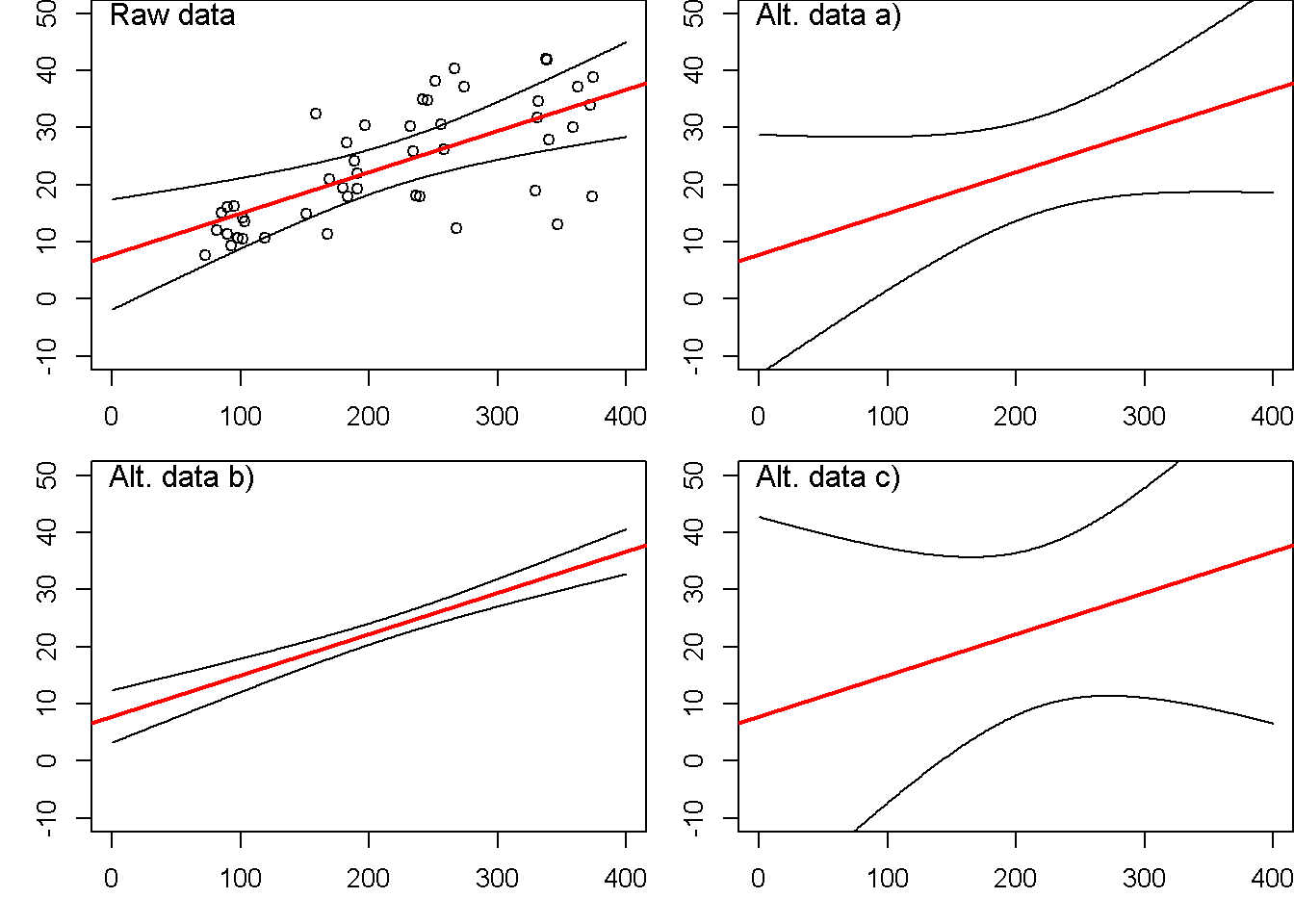Question 26) In the plot above, the raw data used previously is plotted with it 95% Confidence Band. Panels a, b and c represent similar regression lines fit to alternative data sets with different sample sizes.

Why do the confidence bands change size? (1 point)

1. When sample sizes are small there are more outliers and confidence bands are big. When sample sizes are large confidence bands ar narrow.
2. When sample sizes are small parameters are not estimated with precision and confidence bands are big. When sample sizes are large parameters can be estimated precisely and confidence bands are narrow.
3. Change in the error bands is not related to sample size and is just due to random error.
4. None of the above
5. All of the above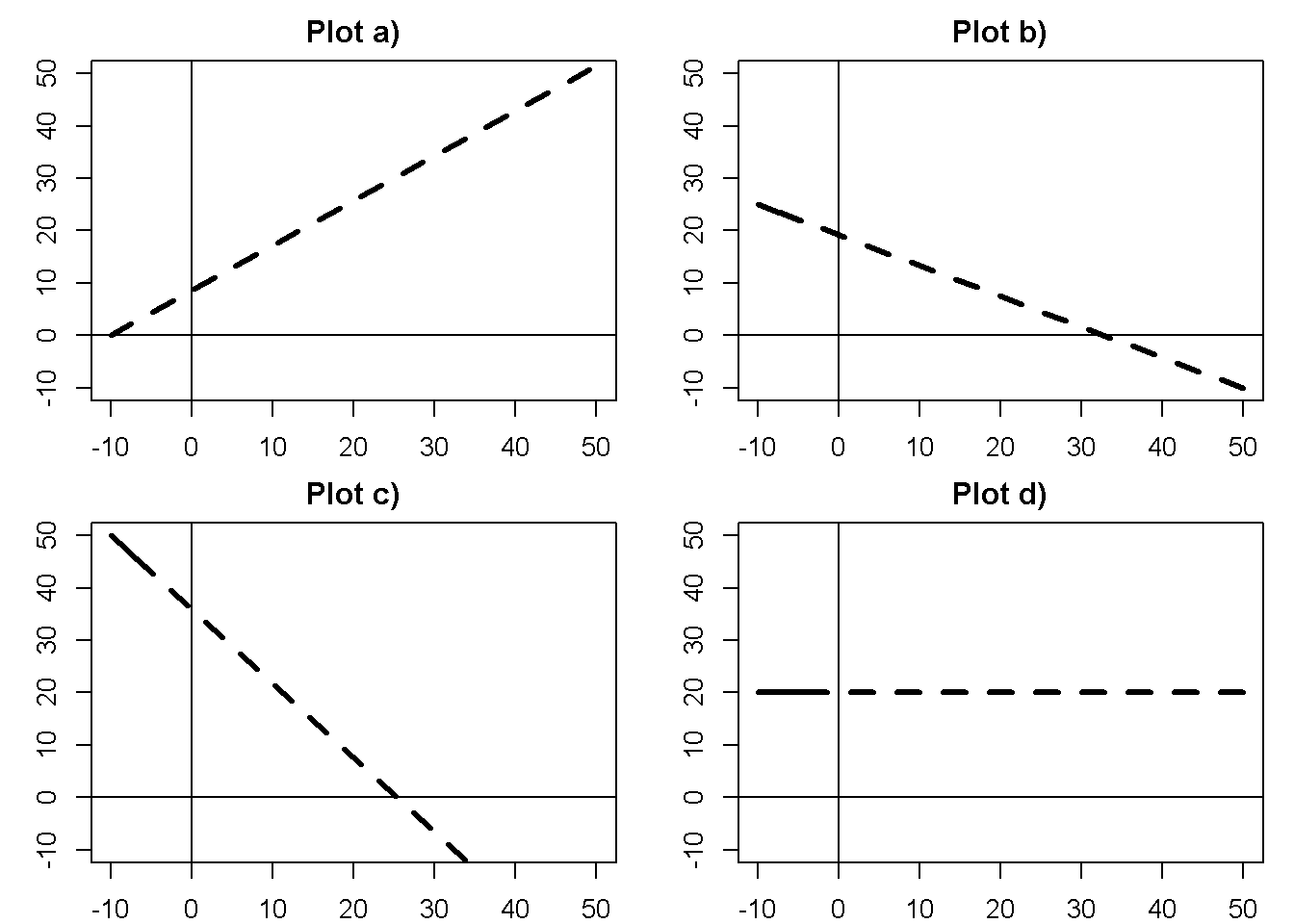Question 27 The plot above shows four hypothetical regression lines with different intercepts and slopes

27a) Which plots have positive (+) slopes? (circle all that apply; 1 point)

1. Plot a)
2. Plot b)
3. Plot c)
4. Plot d)
5. None

27b) Which plots have negative (-) slopes? (circle all that apply; 1 point)

1. Plot a)
2. Plot b)
3. Plot c)
4. Plot d)
5. None

27c) Which plots have zero (0) slopes? (circle all that apply; 1 point)

1. Plot a)
2. Plot b)
3. Plot c)
4. Plot d)
5. None

27d) Which plots have positive intercepts? (circle all that apply; 1 point)

1. Plot a)
2. Plot b)
3. Plot c)
4. Plot d)
5. None

27e) Which plots have negative intercepts? (circle all that apply; 1 point)

1. Plot a)
2. Plot b)
3. Plot c)
4. Plot d)
5. None
##  "Plant"     "Type"      "Treatment" "conc"      "uptake"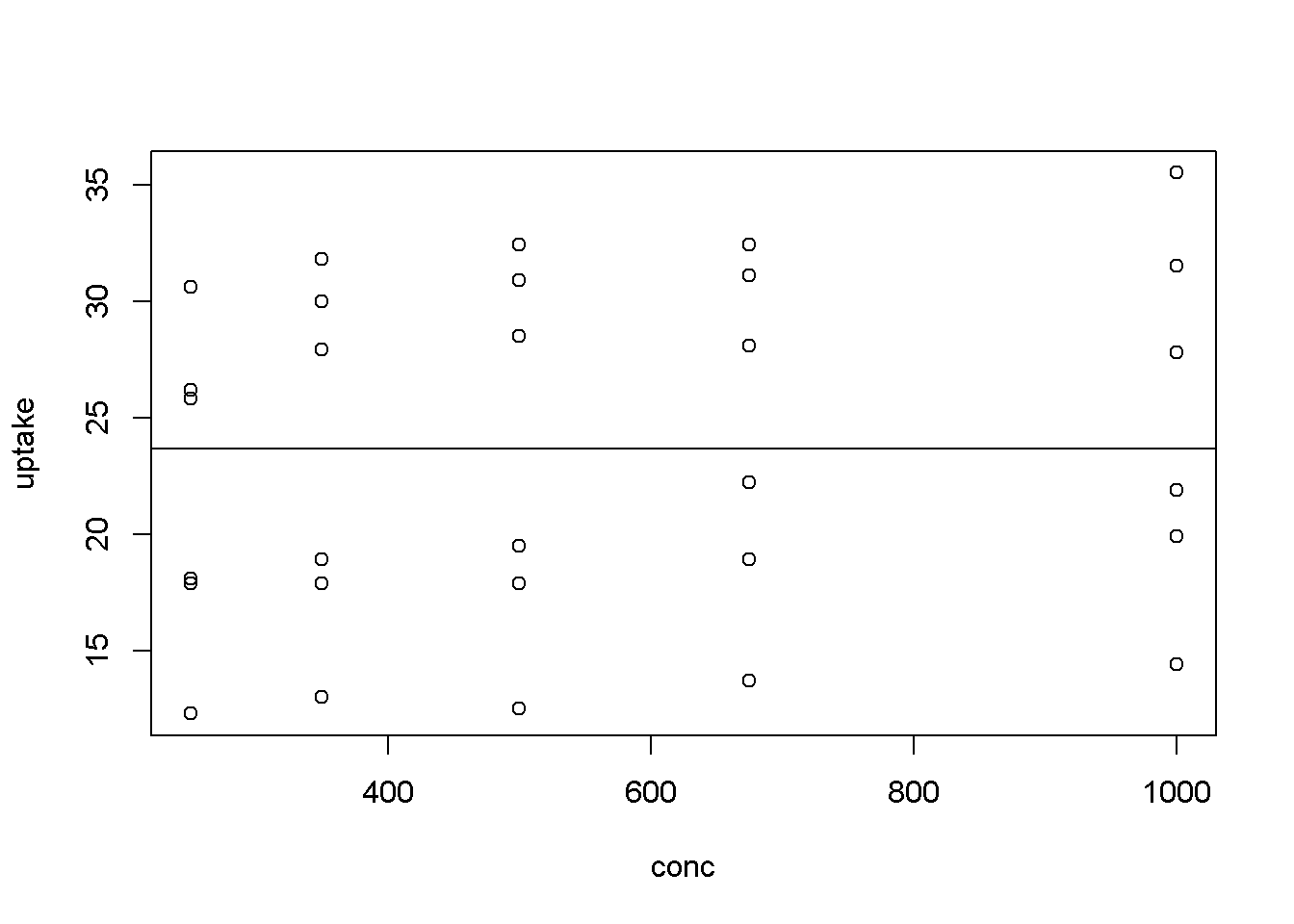Question 28) In a lab experiment researchers were interested in the effect of carbon dioxide concentration (CO2) in the air the rate at which plants can use CO2 for photosynthesis. Their response variable was CO2 uptake rate (“uptake”) and their predictor variable was CO2 concentation (“conc”).

28a) Write the R code to represents a “null” hypothesis (Ho); that is, a model that assumes “update” does not change with “conc” (1 point)

28a) Write the R code to represents a “alternative” hypothesis (Ho); that is, a model that assumes “uptake” does change with “conc” (1 point)

28a) Assume that the null model is called “m.null” and the alternative model is called “m.alt”. Write the one line of R code used to test whether the null hypothesis should be rejected.

anova(m.null,m.alt)
## Analysis of Variance Table
##
## Model 1: uptake ~ 1
## Model 2: uptake ~ conc
##   Res.Df    RSS Df Sum of Sq      F Pr(>F)
## 1     29 1470.7
## 2     28 1436.8  1    33.933 0.6613  0.423
summary(m.alt)
##
## Call:
## lm(formula = uptake ~ conc, data = CO2[i.use, ])
##
## Residuals:
##      Min       1Q   Median       3Q      Max
## -11.0375  -5.1557   0.2152   6.7416  10.0625
##
## Coefficients:
##              Estimate Std. Error t value Pr(>|t|)
## (Intercept) 21.420631   3.037488   7.052 1.14e-07 ***
## conc         0.004017   0.004940   0.813    0.423
## ---
## Signif. codes:  0 '***' 0.001 '**' 0.01 '*' 0.05 '.' 0.1 ' ' 1
##
## Residual standard error: 7.163 on 28 degrees of freedom
## Multiple R-squared:  0.02307,    Adjusted R-squared:  -0.01182
## F-statistic: 0.6613 on 1 and 28 DF,  p-value: 0.423

Question 29 Above is partial R output from an analysis of the data in question 28.

Question 29a) What hypothesis does this data support?

1. Ho
2. Ha

Question 29b) Write a sentence using this output to describe the results of this study.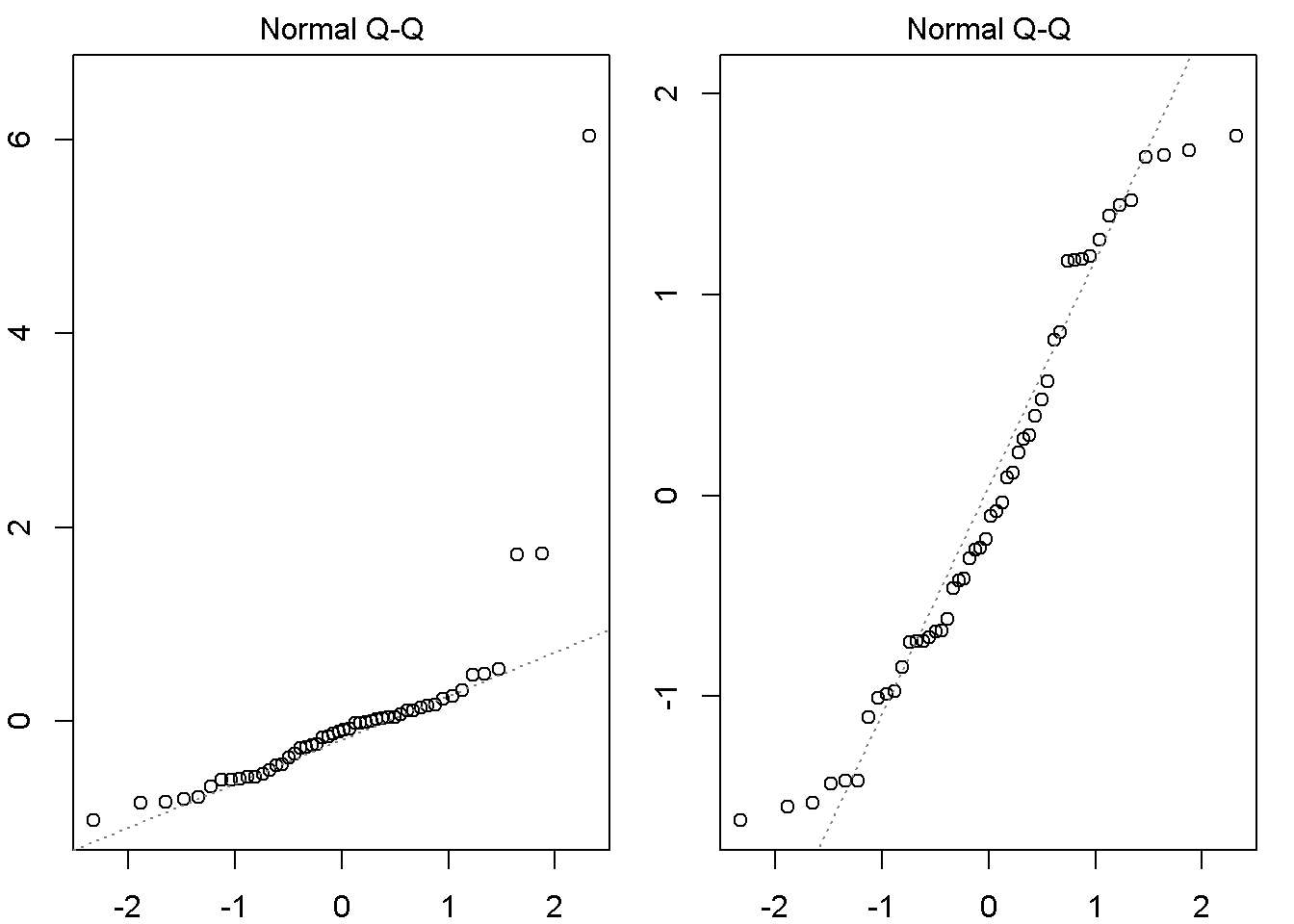Question 30 Which plot indicates a violation of an assumption of regression modeling?

1. The left-hand plot
2. The right-hand plot
3. Neither plot

Question 31 You are reading an old paper from the Journal of Aquatic Ecology and the author’s state “The regression analysis we conducted was highly significant (p < 0.0001), supporting our hypothesis that pH impacted the abundance of insects in southwestern PA streams.”

31a What can you conclude about the slope of the regression model from this p-value?

1. The slope is very likely to be positive (+)
2. The slope is very likely to be negative (-)
3. The slope is very likely to be zero (0)
4. Has a slope that is very likely to be different than zero (0)

31b What can you conclude about the R^2 value of the regression model from this p-value?

1. The R^2 value is very high (near 0.8).
2. The R^2 value is very low (near 0.1).
3. The R^2 value is highly significant.
4. There is no information in this statement about R^2